Next: Stress Tensor in a Up: Mathematical Models of Fluid Previous: General Properties of Stress

# Stress Tensor in a Static Fluid

Consider the surface forces exerted on some infinitesimal cubic volume element of a static fluid. Suppose that the components of the stress tensor are approximately constant across the element. Suppose, further, that the sides of the cube are aligned parallel to the principal axes of the local stress tensor. This tensor, which now has zero non-diagonal components, can be regarded as the sum of two tensors: that is,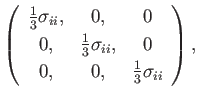(1.12)

and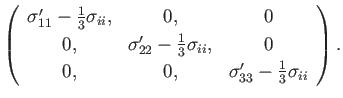(1.13)

The tensor (1.12) is isotropic (see Section B.5), and corresponds to the same normal force per unit area acting inward (because the sign of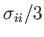is invariably negative) on each face of the volume element. This uniform compression acts to change the element's volume, but not its shape, and can easily be withstood by the fluid within the element.

The tensor (1.13) represents the departure of the stress tensor from an isotropic form. The diagonal components of this tensor have zero sum, in view of Equation (1.11), and thus represent equal and opposite forces per unit area, acting on opposing faces of the volume element, which are such that the forces on at least one pair of opposing faces constitute a tension, and the forces on at least one pair constitute a compression. Such forces necessarily act to change the shape of the volume element, either elongating or compressing it along one of its symmetry axes. Moreover, this tendency cannot be offset by any volume force acting on the element, because such forces become arbitrarily small compared to surface forces in the limit that the element's volume tends to zero (because the ratio of the net volume force to the net surface force scales as the volume to the surface area of the element, which tends to zero in the limit that the volume tends to zero--see Section 1.4). We previously defined a fluid as a material that is incapable of withstanding any tendency of applied forces to change its shape. (See Section 1.2.) It follows that if the diagonal components of the tensor (1.13) are non-zero anywhere inside the fluid then it is impossible for the fluid at that point to be at rest. Hence, we conclude that the principal stresses,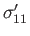,, and, must be equal to one another at all points in a static fluid. This implies that the stress tensor takes the isotropic form (1.12) everywhere in a stationary fluid. Furthermore, this is true irrespective of the orientation of the coordinate axes, because the components of an isotropic tensor are rotationally invariant. (See Section B.5.)

Fluids at rest are generally in a state of compression, so it is convenient to write the stress tensor of a static fluid in the form(1.14)

where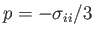is termed the static fluid pressure, and is generally a function of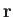and. It follows that, in a stationary fluid, the force per unit area exerted across a plane surface element with unit normal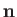is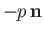. [See Equation (1.3).] Moreover, this normal force has the same value for all possible orientations of. This well-known result--namely, that the pressure is the same in all directions at a given point in a static fluid--is known as Pascal's law, after its discoverer Blaise Pascal (1623-1662), and is a direct consequence of the fact that a fluid element cannot withstand shear stresses, or, alternatively, any tendency of applied forces to change its shape.Next: Stress Tensor in a Up: Mathematical Models of Fluid Previous: General Properties of Stress
Richard Fitzpatrick 2016-03-31